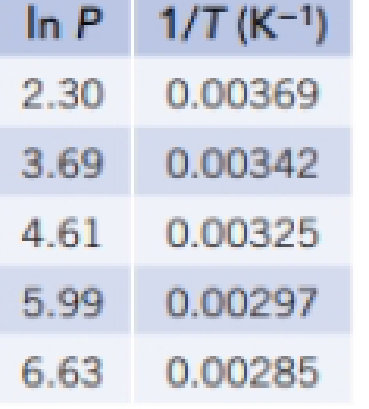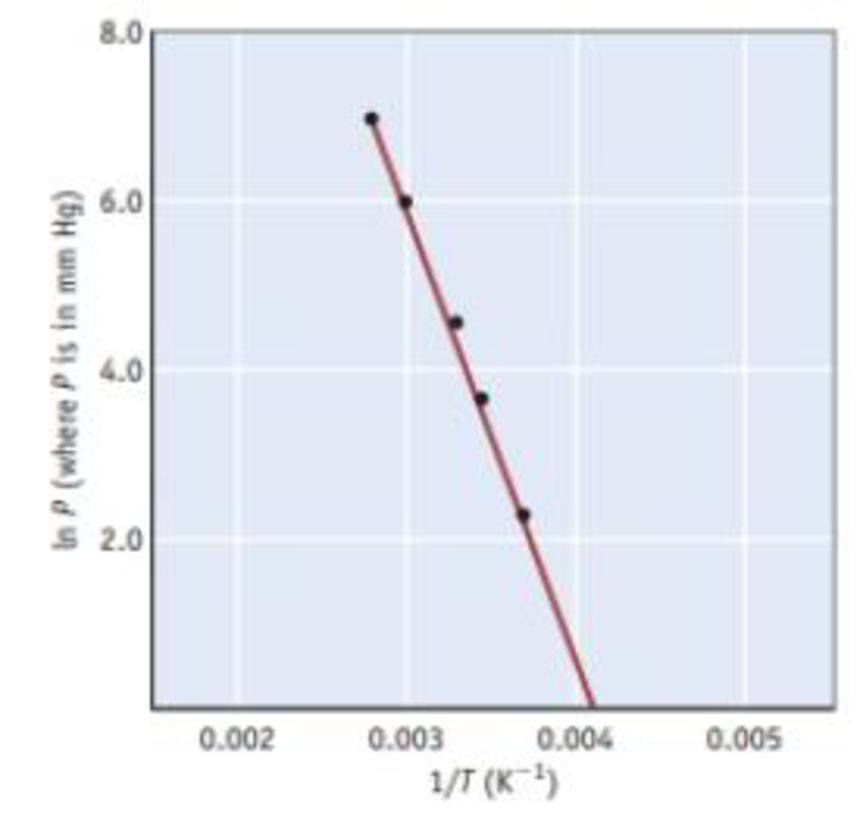Chapter 11, Problem 66SCQ

Chapter
Section
Textbook Problem

The data in the following table were used to create the graph shown below (P = vapor pressure of ethanol (CH3CH2OH) expressed in mm Hg, T = kelvin temperature).(a) Derive an equation for the straight line in this graph. (b) Describe in words how to use the graph to determine the enthalpy of vaporization of ethanol. (c) Calculate the vapor pressure of ethanol at 0.00 °C and at 100 °C.

(a)

Interpretation Introduction

Interpretation:

The equation for the straight line using the given data has to be written.

Concept Introduction:

Clausius-Clapeyron equation:

lnP=(ΔvapH0RT)+C

From this relationship we can calculate the molar enthalpy of vaporization by knowing the corresponding temperature and pressure values.

If we have pressures at two different temperatures, then enthalpy of vaporization can be calculated by

lnP2p1=-ΔvapH0R[1T2-1T1]

Boiling point of a liquid: The temperature at which external pressure and vapour pressure of the liquid become same.

Normal boiling point: When the external pressure is 760mmHg we can call it as normal boiling point.

Explanation

Given:

 ln P 1/T 2.30 0.00369 3.69 0.00342 4.61 0.00325 5.99 0.00297 6.63 0.00285

From the given data we can derive the equation for straight line y=mx+c

Slope=m=ΔvapH0R

Slope=y2-y1x2-x1=5.99-4.610.00297-0

(b)

Interpretation Introduction

Interpretation:

The way by which enthalpy of vaporization can be calculated using the graph has to be given.

Concept Introduction:

Clausius-Clapeyron equation:

lnP=(ΔvapH0RT)+C

From this relationship we can calculate the molar enthalpy of vaporization by knowing the corresponding temperature and pressure values.

If we have pressures at two different temperatures, then enthalpy of vaporization can be calculated by

lnP2p1=-ΔvapH0R[1T2-1T1]

Boiling point of a liquid: The temperature at which external pressure and vapour pressure of the liquid become same.

Normal boiling point: When the external pressure is 760mmHg we can call it as normal boiling point.

(c)

Interpretation Introduction

Interpretation:

The vapour pressure of ethanol at 0°Cand10°C has to be calculated.

Concept Introduction:

Clausius-Clapeyron equation:

lnP=(ΔvapH0RT)+C

From this relationship we can calculate the molar enthalpy of vaporization by knowing the corresponding temperature and pressure values.

If we have pressures at two different temperatures, then enthalpy of vaporization can be calculated by

lnP2p1=-ΔvapH0R[1T2-1T1]

Boiling point of a liquid: The temperature at which external pressure and vapour pressure of the liquid become same.

Normal boiling point: When the external pressure is 760mmHg we can call it as normal boiling point.

Still sussing out bartleby?

Check out a sample textbook solution.

See a sample solution

The Solution to Your Study Problems

Bartleby provides explanations to thousands of textbook problems written by our experts, many with advanced degrees!

Get Started